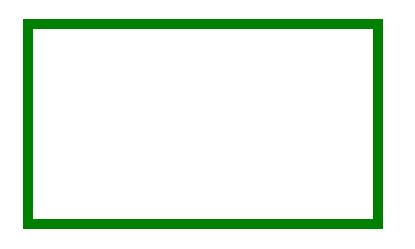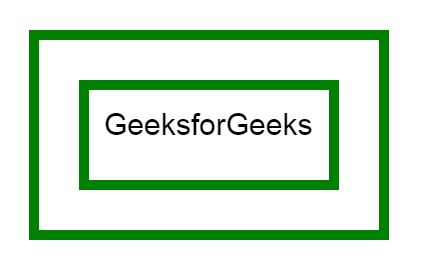HTML | canvas rect() Method

The rect() method in HTML is used to create a rectangle in HTML.

Syntax:

`context.rect(x, y, width, height)`

Parameters:

• x: It stores the x-coordinate of the top-left corner of the rectangle.
• y: It stores the y-coordinate of the top-left corner of the rectangle.
• width: It stores the width in pixel.
• height: It stores the height in pixel.

Example-1:

 ` ` `<``html``> ` ` `  `<``head``> ` `    ``<``title``> ` `        ``HTML canvas rect() Method ` `    `` ` ` ` ` `  `<``body``> ` `    ``<``canvas` `id``=``"GFG"`  `            ``width``=``"500"`  `            ``height``=``"300"``> ` `  `` ` ` `  `    ``<``script``> ` `        ``var x =  ` `            ``document.getElementById("GFG"); ` `        ``var contex =  ` `            ``x.getContext("2d"); ` `             `  `        ``// Create rectangle ` `        ``contex.rect(50, 50, 350, 200); ` `        ``contex.strokeStyle = "green"; ` `        ``contex.lineWidth = "10"; ` `        ``contex.stroke(); ` `    `` ` ` `  ` ` ` `  ` `

Output:Example-2:

 ` ` `<``html``> ` ` `  `<``head``> ` `    ``<``title``> ` `        ``HTML canvas rect() Method ` `    `` ` ` ` ` `  `<``body``> ` `    ``<``canvas` `id``=``"GFG"`  `            ``width``=``"500"`  `            ``height``=``"300"``> ` `  `` ` ` `  `    ``<``script``> ` `        ``var x =  ` `            ``document.getElementById("GFG"); ` `        ``var contex =  ` `            ``x.getContext("2d"); ` `        ``contex.rect(50, 50, 350, 200); ` ` `  `        ``var x = document.getElementById("GFG"); ` `        ``var contex = x.getContext("2d"); ` `        ``contex.rect(100, 100, 250, 100); ` `        ``contex.strokeStyle = "green"; ` `        ``contex.lineWidth = "10"; ` `        ``contex.font = "30px Arial"; ` `        ``contex.fillText("GeeksforGeeks", 120, 150); ` `        ``contex.stroke(); ` `    `` ` ` ` ` `  ` `

Output:Supported Browsers:

• Internet Explorer 9.0
• Firefox
• Safari
• Opera

My Personal Notes arrow_drop_upCheck out this Author's contributed articles.

If you like GeeksforGeeks and would like to contribute, you can also write an article using contribute.geeksforgeeks.org or mail your article to contribute@geeksforgeeks.org. See your article appearing on the GeeksforGeeks main page and help other Geeks.

Please Improve this article if you find anything incorrect by clicking on the "Improve Article" button below.

Article Tags :
Practice Tags :

Be the First to upvote.

Please write to us at contribute@geeksforgeeks.org to report any issue with the above content.